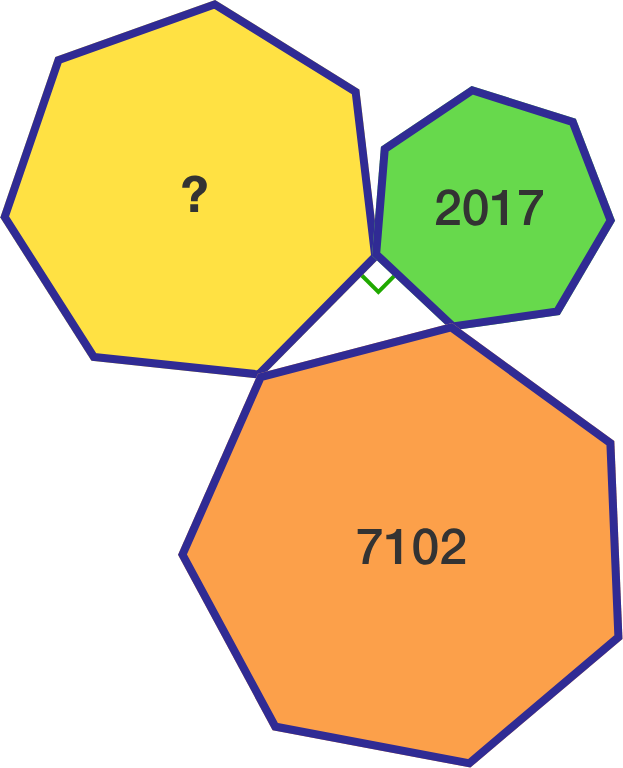# Formula of the area of heptagon?

Geometry Level 3Three regular heptagons with different side lengths form a right triangle, using one side of each. The smallest heptagon has area $2017 \text{ cm}^2,$ while the largest one has area $7102 \text{ cm}^2.$

Find the area of the remaining heptagon in $\text{cm}^2.$

×